# Algorithms for Animation

### Simple formulas to activate your UICourtney Hemphill courtney@carbonfive.com @chemphill #fluentconf
Motion perception
Animations in Interface Design
Formulas as Foundations
Frameworks

## Dev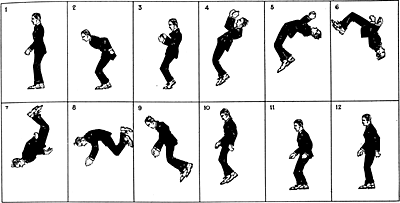## Movement creates life

Gertie the Dinosaur (Winsor McCay, 1914)

## Motion detectionhttp://www.smashingmagazine.com/2013/10/23/smart-transitions-in-user-experience-design/http://www.smashingmagazine.com/2013/10/23/smart-transitions-in-user-experience-design/

## Progressive DisclosureColin Garven
https://dribbble.com/ColinGarven

## Context## Math## Moving pixels

```var ball = document.getElementById('ball');
var start = 0;

var basicAnimation = function (e) {
start += 12;
basic.style.left = start + "px";
if (Math.abs(start) <= 800) { requestAnimationFrame(basicAnimation); }
}
```

## The basics of animation: interpolation

`valueAtTime = (end - start) * time / duration + start`

## Breaking it down to [0-1]

`valueAtTime = (end - start) * time / duration + start`

change = end - start

percent complete = time/duration

## Timing

```var time, startTime;

var start = function () {
startTime = new Date().getTime();
run();
}

var run = function () {
time = new Date().getTime() - startTime;
div.style.left = 900 * time / 1000 + "px";
if(value < 1) requestAnimationFrame(run);
}
```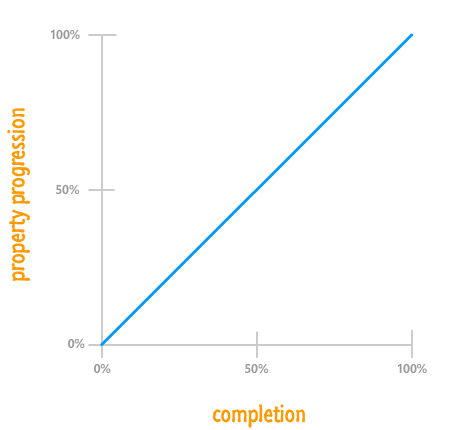```//valueAtTime = (end - start) * time / duration + start
div.style.left = 900-0 * time / 1000 + 0 + "px";
```

"Using a term like nonlinear science is like referring to the bulk of zoology as the study of non-elephant animals."

- Stanislaw Ulam

## Natural movement

Torque, drag, spin, friction

## Easing functions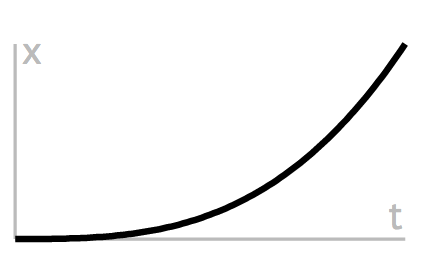## Easing

```			valueAtTime = (end - start) * easingfunction([0-1]) + start
```

Change in property times (some float) plus beginning value.

## Power Functions - EaseIn```var run = function () {
time = new Date().getTime() - startTime;
div.style.left = 900 * Math.pow(percentChange, 3) + "px";
if(time / duration < 1) requestAnimationFrame(run);
}
```

## Power Functions - EaseOut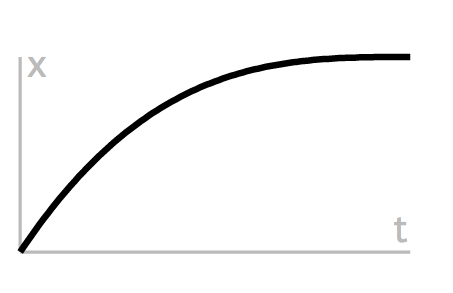```var run = function () {
time = new Date().getTime() - startTime;
div.style.left=(endX - startX)* (1 - Math.pow(1 - (t / d), 3)) +startX+"px";
if(time / duration < 1) requestAnimationFrame(run);
}
```

## Trig! ... sine :)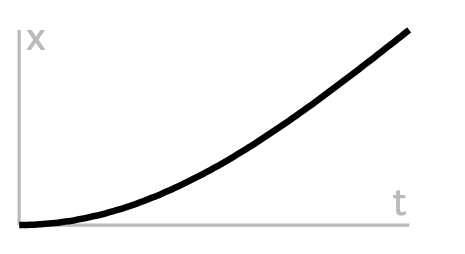```var run = function () {
time = new Date().getTime() - startTime;
div.style.left=(endX - startX)* Math.sin( t/d * Math.PI / 2 ) +startX+"px";
if(time / duration < 1) requestAnimationFrame(run);
}
```

> 1

## Elasticity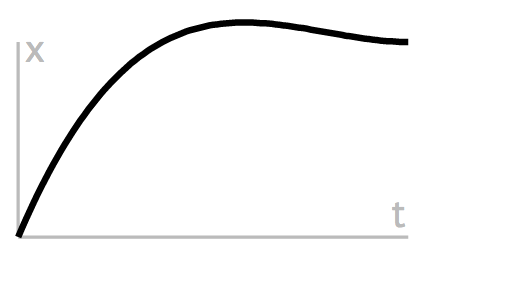```var run = function () {
time = new Date().getTime() - startTime;
div.style.left=(endX - startX)* k * k * ( ( s + 1 ) * k - s ) +startX+"px";
if(time / duration < 1) requestAnimationFrame(run);
}
```

## Bounce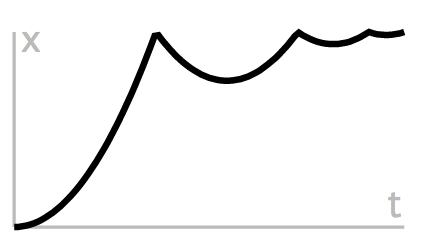```var easeFunc = function(k) {
if ( k < ( 1 / 2.75 ) ) {
return 7.5625 * k * k;
} else if ( k < ( 2 / 2.75 ) ) {
return 7.5625 * ( k -= ( 1.5 / 2.75 ) ) * k + 0.75;
} else if ( k < ( 2.5 / 2.75 ) ) {
return 7.5625 * ( k -= ( 2.25 / 2.75 ) ) * k + 0.9375;
} else {
return 7.5625 * ( k -= ( 2.625 / 2.75 ) ) * k + 0.984375; } }
div.style.left=(endX - startX)* easeFunc(t/d) +startX+"px";
```
Birds on a Wire

• Flinto
• Keynote
• Quartz

## JS Frameworks

• Framer.js
• Tween.js
• GSAP (Greensock)
• jQuery

## CSS

• Animate.css
• CSSanimate.com/
• SASS/LESS mixins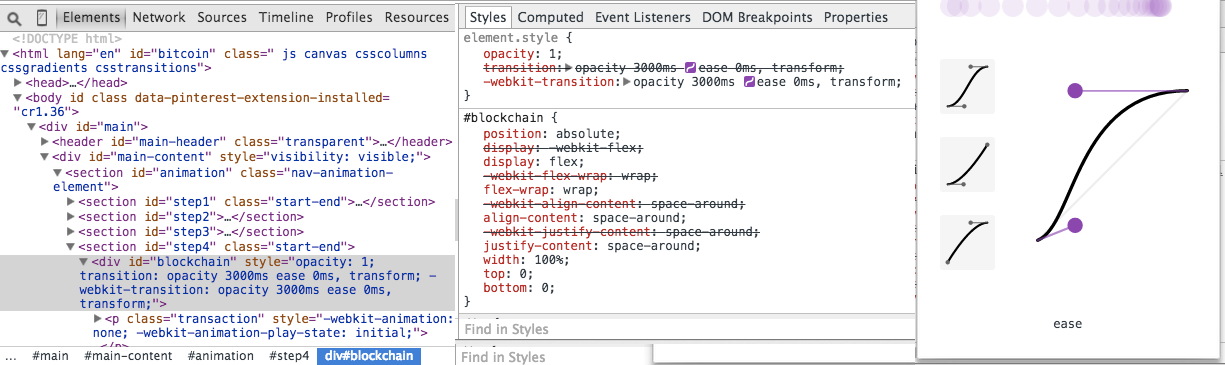## ReferencesCourtney Hemphill
@chemphill
courtney@carbonfive.com
asdfasdf